# Constructing Parabolas with Mathematica

There are several ways to draw a parabola using straight lines. If you get a chance, you should try one sometime - it is always satisfying to see the outline of a curve slowly emerging from a collection of straight lines.

One method uses a set-square. As described in A Book of Curves by E.H. Lockwood (page 3):

Draw a fixed line $$AY$$ and mark a fixed point $$S$$. Place a set square $$UQV$$ (right-angled at $$Q$$) with the vertex $$Q$$ on $$AY$$ and the side $$QU$$ passing through $$S$$ (Fig. 2). Draw the line $$QV$$. When this has been done in a large number of positions, the parabola can be drawn freehand, touching each of the lines so drawn. The curve is said to be the envelope of the variable line $$QV$$ (Fig. 1).

I thought it would be fun to reproduce this method in Mathematica. I’ll work through the construction step-by-step. It is a nice introduction to some of Mathematica’s graphics options.

I’ll start with the points $$A$$ and $$S$$. For simplicity I’ll put $$A$$ at the origin, and choose $$S$$ to be $$(1/7, 1/7)$$.

a = {0, 0};
s = {1/7, 1/7};
Graphics[{
{PointSize[Large], Red, Point[a]},
{PointSize[Large], Red, Point[s]}
},
PlotRange -> {{-1, 1}, {-1, 1}},
Frame -> True]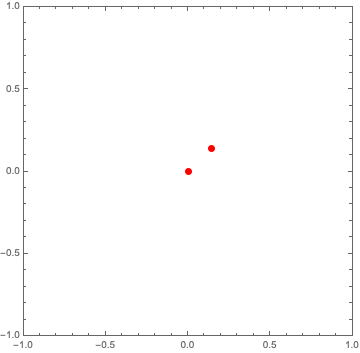Next comes the line $$AY$$. One way to draw this line is to calculate the slope of the line $$AS$$, take the negative reciprocal to get the slope of $$AY$$, and pass the point $$A$$ and the slope to Mathematica’s InfiniteLine. The slope is just $$-S_x/S_y$$, which in this specific case comes out to $$-1$$.

a = {0, 0};
s = {1/7, 1/7};
slope = -s[]/s[];
Graphics[{
{PointSize[Large], Red, Point[a]},
{PointSize[Large], Red, Point[s]},
{Blue, Thick, InfiniteLine[a, {1, slope}]}
},
PlotRange -> {{-1, 1}, {-1, 1}},
Frame -> True]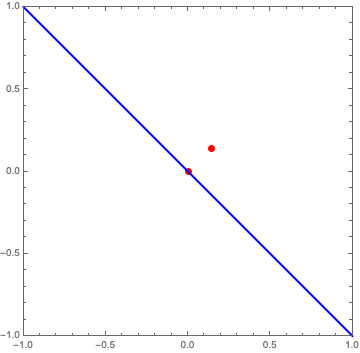Now we need a number of points along $$AY$$ (the blue line above) on which to draw the lines $$QV$$. I would like to control both the number of points and the point spacing, so I’ll introduce both of these as variables: numberOfPoints and pointSpacing. Multiplying these together gives a distance travelled along the line $$AY$$. Moving in one direction from the origin by this distance, we’ll end up at the point:

$$(\text{xEnd}, \text{yEnd}) = \left( \frac{d}{\sqrt{1 + m^2}}, \frac{d m}{\sqrt{1 + m^2}} \right) ,$$

where $$d$$ is the distance and $$m$$ is the slope. Then we can just calculate the step sizes for $$x$$ and $$y$$, and iterate by this step size until we get to the endpoint. In code form:

distance = numberOfPoints*pointSpacing;
xEnd = distance/Sqrt[1 + slope^2];
xStep = xEnd/numberOfPoints;
yEnd = xEnd*slope;
yStep = yEnd/numberOfPoints;
points = Table[{i*xStep, i*yStep}, {i, 1, numberOfPoints}];


Doing the same for the other direction from the origin, and drawing the points:

a = {0, 0};
s = {1/7, 1/7};
slope = -s[]/s[];
numberOfPoints = 10;
pointSpacing = 0.1;
distance = numberOfPoints*pointSpacing;
xEnd = distance/Sqrt[1 + slope^2];
xStep = xEnd/numberOfPoints;
yEnd = xEnd*slope;
yStep = yEnd/numberOfPoints;
allPoints = Join[
Table[{i*xStep, i*yStep}, {i, 1, numberOfPoints}],
Table[{-i*xStep, -i*yStep}, {i, 1, numberOfPoints}]];
Graphics[{
{PointSize[Large], Red, Point[a]},
{PointSize[Large], Red, Point[s]},
{Blue, Thick, InfiniteLine[a, {1, slope}]},
{PointSize[Large], Point[allPoints]}
},
PlotRange -> {{-1, 1}, {-1, 1}},
Frame -> True]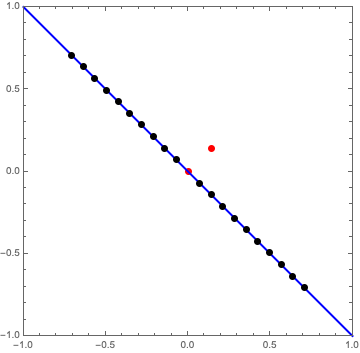Note that we end up with a total of 2*numberOfPoints.

Now, at each of these points $$Q$$, we need a line that is through the point and perpendicular to the line $$QS$$. I’ll construct these lines the same way that I constructed the blue line $$AY$$: calculate the slope as the negative reciprocal of the slope of $$QS$$, and pass the point $$Q$$ and the slope to InfiniteLine. This is probably the most Mathematica-flavored part of the code - I use Map in the shorthand /@ to generate a list of slopes, and MapThread to make the lines.

a = {0, 0};
s = {1/7, 1/7};
slope = -s[]/s[];
numberOfPoints = 10;
pointSpacing = 0.1;
distance = numberOfPoints*pointSpacing;
xEnd = distance/Sqrt[1 + slope^2];
xStep = xEnd/numberOfPoints;
yEnd = xEnd*slope;
yStep = yEnd/numberOfPoints;
allPoints = Join[
Table[{i*xStep, i*yStep}, {i, 1, numberOfPoints}],
Table[{-i*xStep, -i*yStep}, {i, 1, numberOfPoints}]];
getSlope[point_] := -(s[] - point[])/(s[] - point[]);
allSlopes = getSlope /@ allPoints;
newline[point_, slope_] := InfiniteLine[point, {1, slope}];
Graphics[{
{PointSize[Large], Red, Point[a]},
{PointSize[Large], Red, Point[s]},
{Blue, Thick, InfiniteLine[a, {1, slope}]},
{PointSize[Large], Point[allPoints]},
},
PlotRange -> {{-1, 1}, {-1, 1}},
Frame -> True]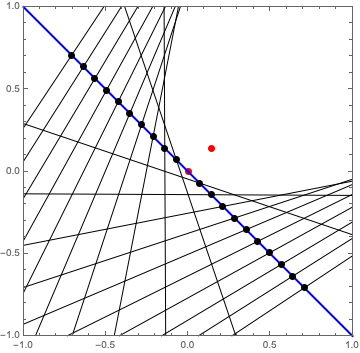Ok, that is starting to look like a parabola!

To make things easier, I’ll gather everything so far up into a function (and stop explicitly showing the points $$Q$$):

parabola[s_, numberOfPoints_, pointSpacing_] := Module[{},
a = {0, 0};
slope = -s[]/s[];
distance = numberOfPoints*pointSpacing;
xEnd = distance/Sqrt[1 + slope^2];
xStep = xEnd/numberOfPoints;
yEnd = xEnd*slope;
yStep = yEnd/numberOfPoints;
allPoints = Join[
Table[{i*xStep, i*yStep}, {i, 1, numberOfPoints}],
Table[{-i*xStep, -i*yStep}, {i, 1, numberOfPoints}]];
getSlope[point_] := -(s[] - point[])/(s[] - point[]);
allSlopes = getSlope /@ allPoints;
newline[point_, slope_] := InfiniteLine[point, {1, slope}];
Graphics[{
{PointSize[Large], Red, Point[a]},
{PointSize[Large], Red, Point[s]},
{Blue, Thick, InfiniteLine[a, {1, slope}]},
},
PlotRange -> {{-1, 1}, {-1, 1}},
Frame -> True]]


Then parabola[{1/7, 1/7}, 100, 0.05] gives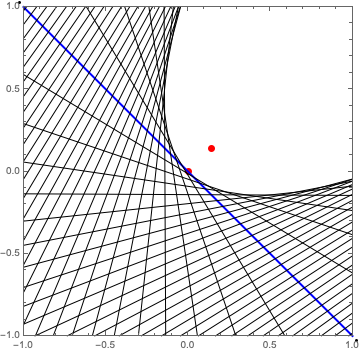Beautiful!

Getting rid of the Frame -> True part and removing all of the graphics except for the final lines lets us generate a bunch of random parabolas this way:

GraphicsGrid[
Partition[
Table[
parabola[{
RandomChoice[{-1, 1}]/RandomReal[{2, 15}],
RandomChoice[{-1, 1}]/RandomReal[{2, 15}]}, 50, 0.05],
{16}], 4],
Frame -> All]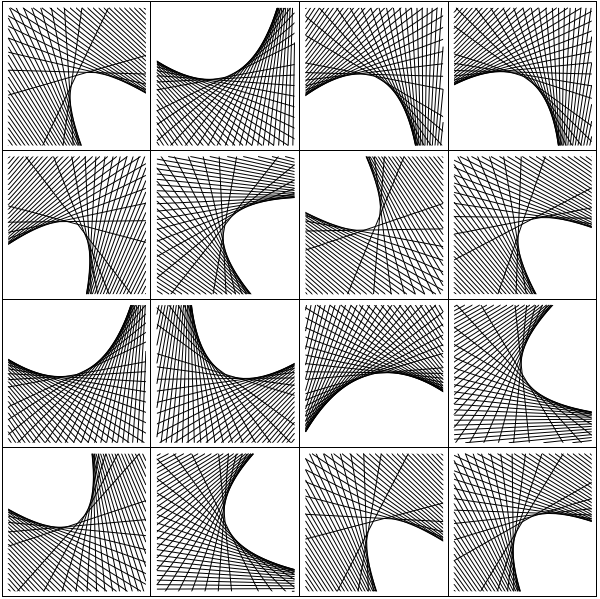##### Landon Lehman
###### Data Scientist

My research interests include data science, statistics, physics, and applied math.